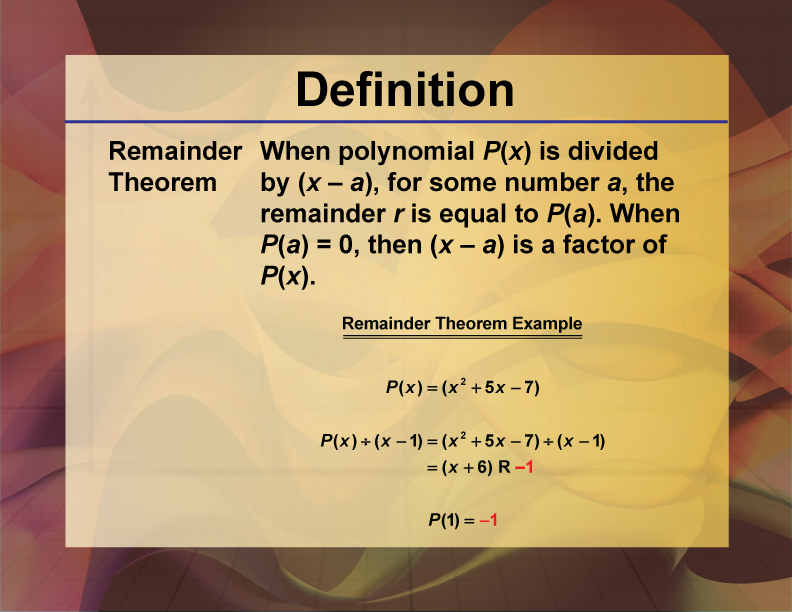## Remainder Theorem, Definition, Formula and Examples

The remaining theorem is a formula for calculating the remainder when dividing a polynomial by a linear polynomial. The amount of items left over after dividing a specific number of things into groups with an equal number of things in each group is known as…